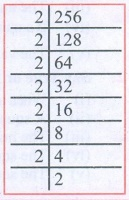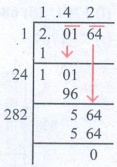Home | | Maths 8th Std | Exercise 1.4 (Square Root)

# Exercise 1.4 (Square Root)

8th Maths : Chapter 1 : Numbers : Square Root: Exercise 1.4 : Text Book Back Exercises Questions with Answers, Solution

Exercise 1.4

1. Fill in the blanks:

(i) The ones digit in the square of 77 is___________. [Answer: 9]

(ii) The number of non-square numbers between 242 and 252 is ______. [Answer: 48]

(iii) The number of perfect square numbers between 300 and 500 is ______. [Answer: 5]

(iv) If a number has 5 or 6 digits in it, then its square root will have ___________ digits. [Answer: 3]

(v) The value of √180 lies between integers ______ and ______. [Answer: 13, 14]

2. Say True or False:

(i) When a square number ends in 6, its square root will have 6 in the unit’s place. [Answer: True]

(ii) A square number will not have odd number of zeros at the end. [Answer: True]

(iii) The number of zeros in the square of 91000 is 9. [Answer: False]

(iv) The square of 75 is 4925. [Answer: False]

(v) The square root of 225 is 15. [Answer: True]

3. Find the square of the following numbers.

(i) 17 (ii) 203 (iii) 1098

Solution:4. Examine if each of the following is a perfect square.

(i) 725 (ii) 190 (iii) 841 (iv) 1089

(i) 725 (ii) 190 (iii) 841 (iv) 1089

Solution:

(i) 725725 = 5 × 5 × 29 = 52 × 29

Here the second prime factor 29 does not have a pair.

Hence 725 is not a perfect square number.

(ii) 190190 = 2 × 5 × 19

Here the factors 2, 5 and 9 does not have pairs.

Hence 190 is not a perfect square number.

(iii) 841

841 = 29 × 29

Hence 841 is a perfect square

(iv) 10891089 = 3 × 3 × 11 × 11

1089 = 32 × 112

√1089 = 3 × 11 = 33

Hence 1089 is a perfect square

5. Find the square root by prime factorisation method.

(i) 144 (ii) 256 (iii) 784 (iv) 1156 (v) 4761 (vi) 9025

Solution:

(i) 144144 = 2 × 2 × 2 × 2 × 3 × 3

√l44 = 2 × 2 × 3 = 12

(ii) 256256 = 2 × 2 × 2 × 2 × 2 × 2 × 2 × 2

√256 = 2 × 2 × 2 × 2 = 16

(iii) 784784 = 2 × 2 × 2 × 2 × 7 × 7

√784 = 2 × 2 × 2 × 2 × 7 × 7 = 28

(iv) 11561156 = 2 × 2 × 17 × 17

1156 = 22 × 172

1156 = (2 × 17)2

√1156 = √(2 × l7)2 = 2 × 17 = 34

√1156 = 34

(v) 47614761 = 3 × 3 × 23 × 23

4761 = 32 × 232

4761 = (3 × 23)2

√4761 = √(3 × 23)2

√4761 = 3 × 23

√4761 = 69

(vi) 90259025 = 5 × 5 × 19 × 19

9025 = 52 × 192

9025  = (5 × 19)2

√925 = = √ (5 × 19)2 = 5 × 19 = 95

6. Find the square root by long division method.

(i) 1764 (ii) 6889 (iii) 11025 (iv) 17956 (v) 418609

Solution:7. Estimate the value of the following square roots to the nearest whole number:

(i) √440 (ii) √800 (iii) √1020

Solution:

(i) √440

We have 202 = 400

212 = 441

√440 21

(ii) √800

We have 282 = 784

292 = 841

√800 28

(iii) √1020

We have 312 = 961

322 = 1024

√1020 32.

8. Find the square root of the following decimal numbers and fractions.

(i) 2.89 (ii) 67.24 (iii) 2.0164 (iv) 144/225 (v)Solution:

(i) 2.89√2.89 = 1.7

(ii) 67.24√67.24 = 8.2

(iii) 2.0164√2.0164 = 1.42

(iv) 144/225 = √[144/225] = 12/15

(v) 7 (18/49) = √[361/49] = √361/√49 = √192/√72 =19/79. Find the least number that must be subtracted to 6666 so that it becomes a perfect square. Also, find the square root of the perfect square thus obtained.

Solution:

Let us work out the process of finding the square root of 6666 by long division method.The remainder in the last step is 105. Is if 105 be subtracted from the given number the remainder will be zero and the new number will be a perfect square.

The required number is 105. The square number is 6666 − 105 = 6561.

√6561 = 81

10. Find the least number by which 1800 should be multiplied so that it becomes a perfect square. Also, find the square root of the perfect square thus obtained.

Solution:We find 1800 = 2 × 2 × 3 × 3 × 5 × 5 × 2

= 22 × 32 × 52 × 2

Here the last factor 2 has no pair. So if we multiply

1800 by 2, then the number becomes a perfect square.

1800 × 2 = 3600 is the required perfect square number.

3600 = 1800 × 2

3600 = 22 × 32 × 52 × 2 × 2

3600 = 22 × 32 × 52 × 22

= (2 × 3 × 5 × 2)2

√3600 = √(2 × 3 × 5 × 2)2

= 2 × 3 × 5 × 2 = 60

√3600 = 60.

Objective Type Questions

11. The square of 43 ends with the digit ______.

(A) 9

(B) 6

(C) 4

(D) 3

Solution: Ones digit = 3 × 3 = 9

12. _______ is added to 242 to get 252.

(A) 42

(B) 52

(C) 62

(D) 72

Solution:

252 = 25 × 25 = 625

242 = 24 × 24 = 576

625 −576 = 49

49 = 72

13. √48 is approximately equal to ______.

(A) 5

(B) 6

(C) 7

(D) 8

Solution: √49 = 7

14. √128 - √98 + √18 = ______.

(A) √2

(B) √8

(C) √48

(D) √32

15. The number of digits in the square root of 123454321 is ______.

(A) 4

(B) 5

(C) 6

(D) 7

Solution:

= [n + 1] / 2 = 10/2 = 5

Exercise 1.4

1. (i) 9 (ii) 48 (iii) 5 (iv) 3 (v) 13, 14

2. (i) True (ii) True (iii) False (iv) False (v) True

3. (i) 289 (ii) 41209 (iii) 1205604

4. (i) No (ii) No (iii) Yes (iv) Yes

5. (i) 12 (ii) 16 (iii) 28 (iv) 34 (v) 69 (vi) 95

6. (i) 42 (ii) 83 (iii) 105 (iv) 134 (v) 647

7. (i) 21 (ii) 28 (iii) 32

8. (i) 1.7 (ii) 8.2 (iii) 1.42 (iv) 12/15 (v) 2 5/7

9. 105, 81 10. 2, 60

11. (A) 9

12. (D) 72

13. (C) 7

14. (D) √32

15. (B) 5

Tags : Questions with Answers, Solution | Numbers | Chapter 1 | 8th Maths , 8th Maths : Chapter 1 : Numbers
Study Material, Lecturing Notes, Assignment, Reference, Wiki description explanation, brief detail
8th Maths : Chapter 1 : Numbers : Exercise 1.4 (Square Root) | Questions with Answers, Solution | Numbers | Chapter 1 | 8th Maths